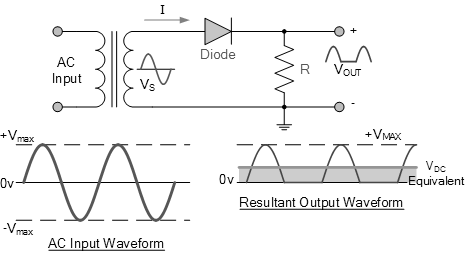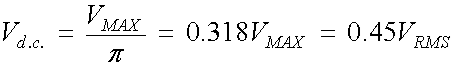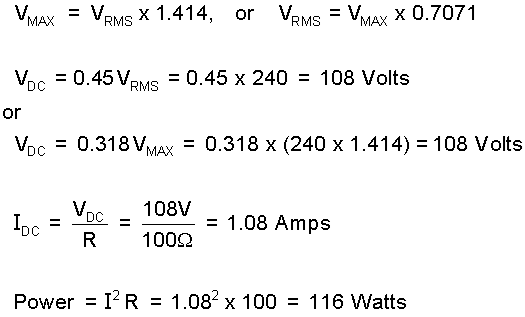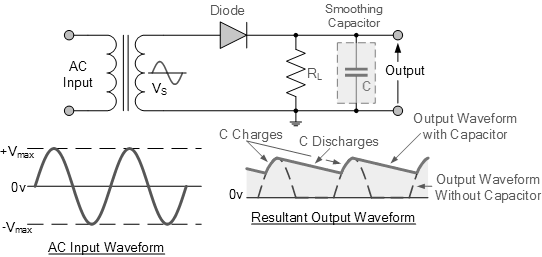# What is Half Wave Rectifier?

A rectifier is a circuit which converts the Alternating Current (AC) input power into a Direct Current (DC) output power. The input power supply may be either a single-phase or a multi-phase supply with the simplest of all the rectifier circuits being that of the Half Wave Rectifier. The power diode in a half wave rectifier circuit passes just one half of each complete sine wave of the AC supply in order to convert it into a DC supply. Then this type of circuit is called a “half-wave” rectifier because it passes only half of the incoming AC power supply as shown below.

Half Wave Rectifier CircuitDuring each “negative” half cycle of the AC sinusoidal input waveform, the diode is reverse biased as the anode is negative with respect to the cathode. Therefore, NO current flows through the diode or circuit. Then in the negative half cycle of the supply, no current flows in the load resistor as no voltage appears across it so therefore, Vout = 0.  The current on the DC side of the circuit flows in one direction only making the circuit Unidirectional. The load resistance from the diode receives positive half of the waveform, zero volt, positive half of the waveform, zero volt, etc., so this irregular voltage value is equal to the equivalent DC voltage of 0.318 * Vmax. Input sine wave, or 0.45 * Vrms sine wave input waveform. Next, calculate the equivalent DC voltage VDC across the load resistance as follows:Here, VMAX is the maximum or peak voltage value of the AC sinusoidal supply, and VRMS is the RMS (Root Mean Squared) value of the supply voltage.

Example:

Calculate the voltage drop VDC and current IDC flowing through a 100Ω resistor connected to a 240Vrms single-phase half-wave rectifier as described above. It also calculates the average DC power consumed by the load.During rectification, the output DC voltage and current are both "ON" and "OFF" in each cycle. Since the voltage across the load resistor is present only for the positive half cycle (50% of the input waveform), this results in a low average DC value being fed to the load. Changing this rectified output waveform between the "ON" and "OFF" states will produce a waveform that exhibits a large amount of "ripple", which is an undesirable characteristic. Forms a DC ripple with a frequency equal to that of the AC power supply. Normally, when rectifying AC voltage, we want to produce a "smooth" and continuous DC voltage, without any voltage fluctuations or ripples. One way to do this is to connect a large value capacitor across the output voltage terminals in parallel with the load resistor as shown below. This type of capacitor is often referred to as a smoothing capacitor.

Half-wave Rectifier with Smoothing CapacitorWhen a rectifier is used to supply a direct voltage (DC) source from an alternating current (AC) source, the amount of ripple voltage can be further reduced by using larger rated capacitors, but There are limitations on both the cost and size of smoothing capacitors. used.  For a given capacitor value, a larger load current (lower load resistance) will discharge the capacitor faster (RC Time Constant) and thus increase the generated ripple. Then, for a single-phase half-wave rectifier circuit using power diodes, it is not very practical to try to reduce the ripple voltage by smoothing the capacitor. In this case, it is more convenient to use "Full Wave Rectification". In practice, half-wave rectifiers are used most often in low-power applications because of their major disadvantages. The output amplitude is less than the input amplitude, there is no output for the negative half cycle, so half the power is wasted and the output is pulsed to DC, resulting in overshoot. To overcome these disadvantages, several power diodes are connected together to create a full wave rectifier.

Prasun Barua

Prasun Barua is an Engineer (Electrical & Electronic) and Member of the European Energy Centre (EEC). His first published book Green Planet is all about green technologies and science. His other published books are Solar PV System Design and Technology, Electricity from Renewable Energy, Tech Know Solar PV System, C Coding Practice, AI and Robotics Overview, Robotics and Artificial Intelligence, Know How Solar PV System, Know The Product, Solar PV Technology Overview, Home Appliances Overview, Tech Know Solar PV System, C Programming Practice, etc. These books are available at Google Books, Google Play, Amazon and other platforms.

*